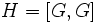# Derived subgroup not is quasiautomorphism-invariant

This article gives the statement, and possibly proof, of the fact that for a group, the subgroup obtained by applying a given subgroup-defining function (i.e., commutator subgroup) does not always satisfy a particular subgroup property (i.e., {{{property}}}
Property "Proves property satisfaction of" (as page type) with input value "{{{property}}}" contains invalid characters or is incomplete and therefore can cause unexpected results during a query or annotation process.
)
View subgroup property satisfactions for subgroup-defining functions$|$ View subgroup property dissatisfactions for subgroup-defining functions

## Statement

It is possible to have a group$G$ such that the commutator subgroup (or derived subgroup)$[G,G]$ is not a quasiautomorphism-invariant subgroup, i.e., there exists a Quasiautomorphism (?)$\sigma$ of$G$ such that$\sigma(H) \ne H$.

## Proof

Further information: inner automorphism group of wreath product of groups of order p

Suppose$p$ is a prime number greater than$3$. Let$G$ be the group isomorphic to the inner automorphism group of the wreath product of two groups of order$p$.$G$ is a group of order$p^p$ with an elementary abelian normal subgroup$N$ of order$p^{p-1}$, an element of order$p$ acting on it from outside, and every non-identity element of$G$ has order$p$.

Consider the commutator subgroup$H = [G,G]$.$H$ is a group of order$p^{p-2}$, contained inside the elementary abelian normal subgroup.

we can construct a quasiautomorphism$\sigma$ of$G$ that does not preserve$H$ as follows: the restriction of$\sigma$ to$N$ is an automorphism of$N$ that fixes the center$Z(G)$ (which is cyclic of order$p$) but does not send$H$ to itself, and$\sigma$ fixes every element of$G$ outside$H$. Note that we need$p > 3$ to ensure that$H$ is strictly bigger than$Z(G)$, which is necessary to be able to construct a$\sigma$ with the desired specifications.